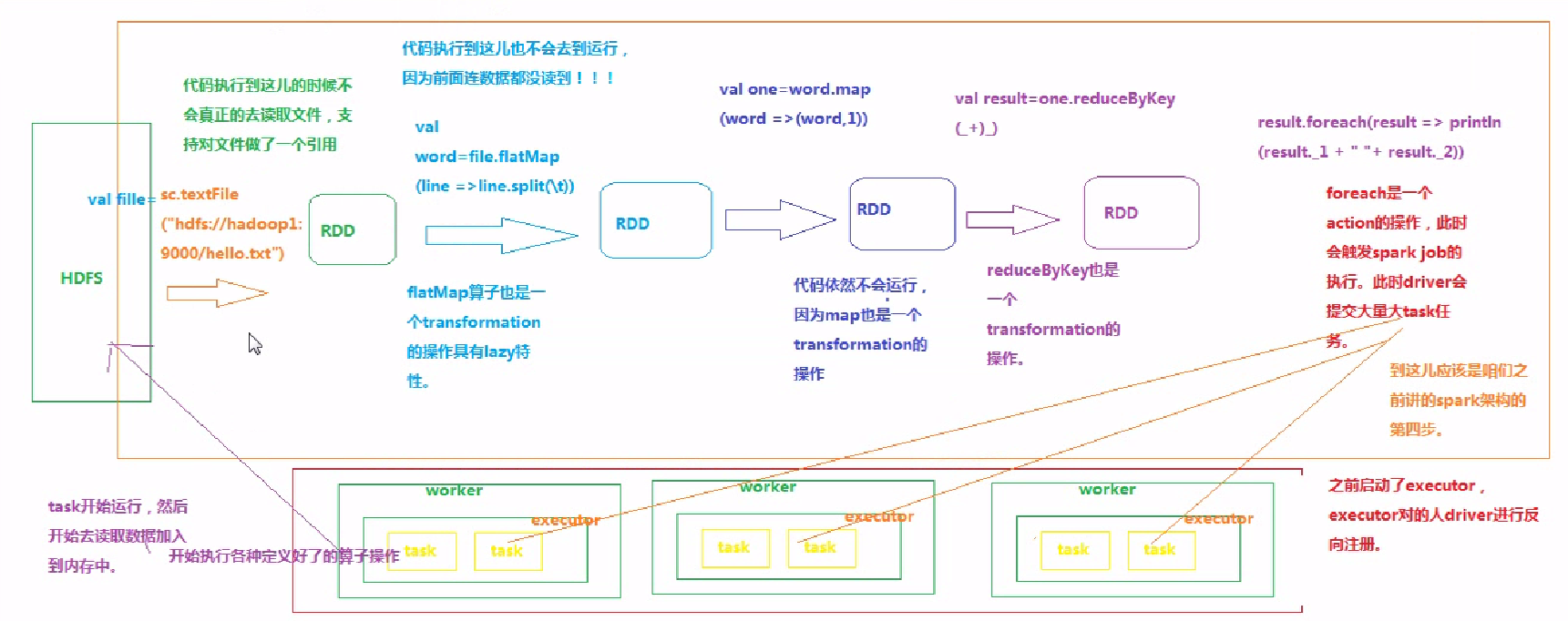# spark 常用函数介绍（python）

## 获取SparkContext

python语法
1. 获取sparkSession：  se = SparkSession.builder.config(conf = SparkConf()).getOrCreate()
2. 获取sparkContext：  sc = se.sparkContext
3. 获取sqlContext：    sq = SparkSession.builder.getOrCreate()
4. 获取DataFrame：     df = sqlContext.createDataFrame(userRows)


## 数据格式

1. [[u'3', u'5'], [u'4', u'6'], [u'4', u'5'], [u'4', u'2']]   拆分或截取的原始数据，  可以通过 map 中的 x, x 来获取对应列的数据
可以通过 map 来转换为key-value 数据格式  例如：  df3 = df2.map(lambda x: (x, x))    //python语法

2. key-value 数据格式
[(u'3', u'5'), (u'4', u'6'), (u'4', u'5'), (u'4', u'2')]   中每一个（） 表示一组数据，  第一个表示key    第二个表示value
3）PipelinedRDD  类型表示   key-value形式数据


## RDD

RDD是Spark中的抽象数据结构类型，任何数据在Spark中都被表示为RDD。从编程的角度来看，RDD可以简单看成是一个数组。和普通数组的区别是，RDD中的数据是分区存储的，这样不同分区的数据就可以分布在不同的机器上，同时可以被并行处理。因此，Spark应用程序所做的无非是把需要处理的数据转换为RDD，然后对RDD进行一系列的变换和操作从而得到结果。

（1）、通过读取本地或者hdfs上的文件创建RDD

//scala语法
path = "hdfs://master:9000/examples/examples/src/main/resources/people.txt"
rdd1 = sc.textFile(path,2)

path = "file:///usr/local/spark/spark-1.6.0-bin-hadoop2.6/README.md"  //local file
rdd1 = sc.textFile(path,2)


（2）通过并行化的方式创建RDD. 其实就是通过我们自己取模拟数据

//scala语法
# list转RDD
sc.parallelize([1,2,3,4,5], 3)  #意思是将数组中的元素转换为RDD，并且存储在3个分区上、[2,3]、[4,5]。如果是4个分区：、、、[4,5]

val str=Array("you jump","I jump")
val list = Array(1,2,3,4,5,6)
val listadd = sc.parallelize(list) 可以看到返回值就是RDD，当然可以调用RDD中的函数，比如reduce算子等
listadd.reduce(_+_)

# rdd转list
list = RDD.collect()


spark的函数主要分两类，Transformations和Actions。Transformations为一些数据转换类函数，actions为一些行动类函数：

transformation 会针对已有的RDD创建一个新的RDD，而action则主要对RDD进行最后的操作。transformation只是记录了对RDD的操作，并不会触发spark程序的执行，只有当transform之后接着一个action操作，那么所有的transformation才会执行。比如

//scala语法
val file=sc.textFile("hdfs://hadoop1:9000/hello.txt").flatMap(line => line.split("\t"))Transformations

map(func [, preservesPartitioning=False]) — 返回一个新的分布式数据集，这个数据集中的每个元素都是经过func函数处理过的。

//python语法
>>> data = [1,2,3,4,5]
>>> distData = sc.parallelize(data).map(lambda x: x+1).collect()
#结果：[2,3,4,5,6]


filter(func) — 返回一个新的数据集，这个数据集中的元素是通过func函数筛选后返回为true的元素（简单的说就是，对数据集中的每个元素进行筛选，如果符合条件则返回true，不符合返回false，最后将返回为true的元素组成新的数据集返回）。

//python语法
>>> rdd = sc.parallelize(data).filter(lambda x:x%2==0).collect()
#结果：[2, 4]


flatMap(func [, preservesPartitioning=False]) — 类似于map(func)， 但是不同的是map对每个元素处理完后返回与原数据集相同元素数量的数据集，而flatMap返回的元素数不一定和原数据集相同。每个输入元素可能被影射为0个或多个输出元素。

//python语法
#### for flatMap()
>>> rdd = sc.parallelize([2,3,4])
>>> sorted(rdd.flatMap(lambda x: range(1,x)).collect())
#结果：[1, 1, 1, 2, 2, 3]
>>> sorted(rdd.flatMap(lambda x:[(x,x), (x,x)]).collect())
#结果：[(2, 2), (2, 2), (3, 3), (3, 3), (4, 4), (4, 4)]

#### for map()
>>> rdd = sc.parallelize([2,3,4])
>>> sorted(rdd.map(lambda x: range(1,x)).collect())
#结果：[, [1, 2], [1, 2, 3]]
>>> sorted(rdd.map(lambda x:[(x,x), (x,x)]).collect())
#结果：[[(2, 2), (2, 2)], [(3, 3), (3, 3)], [(4, 4), (4, 4)]]


mapPartitions(func [, preservesPartitioning=False]) —mapPartitions是map的一个变种。map的输入函数是应用于RDD中每个元素，而mapPartitions的输入函数是应用于每个分区，也就是把每个分区中的内容作为整体来处理的。

//python语法
>>> rdd = sc.parallelize([1,2,3,4,5], 3)
>>> def f(iterator): yield sum(iterator)
>>> rdd.mapPartitions(f).collect()
#结果：[1,5,9]


mapPartitionsWithIndex(func [, preservesPartitioning=False]) —类似于mapPartitions, 只是包含两个参数.第一个参数为分区的索引，第二个参数为每个分区内的元素迭代器。输出是一个包含元素列表的迭代器。

//python语法
>>> rdd = sc.parallelize([1,2,3,4,5], 3)
>>> def f(splitIndex, iterator): yield splitIndex
>>> rdd.mapPartitionsWithIndex(f).collect()
#结果：[0,1,2]   #三个分区的索引


reduceByKey(func [, numPartitions=None, partitionFunc=<function portable_hash at 0x7fa664f3cb90>]) — reduceByKey就是对元素为kv对的RDD中Key相同的元素的value进行reduce，因此，key相同的多个元素的值被reduce为一个值，然后与原RDD中的key组成一个新的kv对。等价于pandas中的groupby

//python语法
>>> from operator import add
>>> rdd = sc.parallelize([("a", 1), ("b", 1), ("a", 1)])
>>> sorted(rdd.reduceByKey(add).collect())
>>> #或者 sorted(rdd.reduceByKey(lambda a,b:a+b).collect())
#结果：[('a', 2), ('b', 1)]


sortByKey([ascending=True, numPartitions=None, keyfunc=<function <lambda> at 0x7fa665048c80>]) 返回排序后的数据集。该函数就是对kv对的RDD数据进行排序，keyfunc是对key进行处理的函数，如非需要，不用管。 也就是说先按keyfunc对key进行处理，然后按照key进行排序。

//python语法
>>> tmp = [('a', 1), ('b', 2), ('1', 3), ('D', 4)]
>>> sc.parallelize(tmp).sortByKey(True, 1).collect()
#结果： [('1', 3), ('D', 4), ('a', 1), ('b', 2)]
>>> sc.parallelize(tmp).sortByKey(True, 2, keyfunc=lambda k:k.lower()).collect()
#结果：[('1', 3), ('a', 1), ('b', 2), ('D', 4)]
#注意，比较两个结果可看出，keyfunc对键的处理只是在数据处理的过程中起作用，不能真正的去改变键名


join(otherDataset [, numPartitions=None]) — join就是对元素为kv对的RDD中key相同的value收集到一起组成(v1,v2)，然后与原RDD中的key组合成一个新的kv对，返回。即RDD1中的元素与RDD2中元素具有相同key值的尝试合并。

//python语法
>>> x = sc.parallelize([("a", 1), ("b", 4)])
>>> y = sc.parallelize([("a", 2), ("a", 3)])
>>> sorted(x.join(y).collect())
#结果：[('a', (1, 2)), ('a', (1, 3))]


cartesian(otherDataset) — 返回一个笛卡尔积的数据集，这个数据集是通过计算两个RDDs得到的。即RDD1中的元素与RDD2中元素任意两个元素尝试合并。

//python语法
>>> x = sc.parallelize([1,2,3])
>>> y = sc.parallelize([4,5])
>>> x.cartesian(y).collect()
#结果：[(1, 4), (1, 5), (2, 4), (2, 5), (3, 4), (3, 5)]


RDD 删除 重复 元素

intRdd.distinct()     #去重


sRdd = stringRdd.randomSplit([0.4,0.6])    将 stringRdd 以4:6 分为2个 RDD，  获取其中一个 RDD 的方法为： sRdd


RDD 中 groupBy 分组计算

//python语法
gRdd = intRdd.groupBy(lambda x: x<2)   #将会分为2组，  访问第一组：  print(sorted(gRdd)),  访问第二组：print(sorted(gRdd))，因为gRdd和gRdd表示默认生成的组名

（1）获取第一组信息： print(gRdd, sorted(gRdd))
（2） 获取第二组信息： print(gRdd, sorted(gRdd))   其中，   前半部分 gRdd 表示获取别名 a


## Action (这里只讲支持python的)

reduce(func) — reduce将RDD中元素两两传递给输入函数，同时产生一个新的值，新产生的值与RDD中下一个元素再被传递给输入函数直到最后只有一个值为止。

>>> from operator import add
>>> sc.parallelize([1,2,3,4,5]).reduce(add)
# 结果：15


collect() — 返回RDD中的数据，以list形式。

>>> sc.parallelize([1,2,3,4,5]).collect()
#结果：[1,2,3,4,5]


count() — 返回RDD中的元素个数。

>>> sc.parallelize([1,2,3,4,5]).count
#结果：5


first() — 返回RDD中的第一个元素。

>>> sc.parallelize([1,2,3,4,5]).first()
#结果：1


take(n) — 返回RDD中前n个元素。

>>> sc.parallelize([1,2,3,4,5]).take(2)
#结果：[1,2]


takeOrdered(n [, key=None]) — 返回RDD中前n个元素，但是是升序(默认)排列后的前n个元素，或者是通过key函数指定后的RDD（这个key我也没理解透，后面在做详解）。等价于sql语句里面 order limit

>>> sc.parallelize([9,7,3,2,6,4]).takeOrdered(3)
#结果：[2,3,4]
>>> sc.parallelize([9,7,3,2,6,4]).takeOrdered(3, key=lambda x:-x)
#结果：[9,7,6]


1） intRdd1.stats() 统计 intRdd1， 结果为：(count: 5, mean: 5.0, stdev: 2.82842712475, max: 9, min: 1) mean表示平均值， stdev 表示标准差
2）intRdd3.min() 最新值，
3）intRdd3.max() 最大值
4）intRdd3.stdev() 标准差
5）intRdd3.count() 数据条数
6）intRdd3.sum() 求和
7）intRdd3.mean() 平均值

saveAsTextFile(path [, compressionCodecClass=None]) — 该函数将RDD保存到文件系统里面，并且将其转换为文本行的文件中的每个元素调用 tostring 方法。path - 保存于文件系统的路径，compressionCodecClass压缩编码类型，如gzip

>>> tempFile = NamedTemporaryFile(delete=True)
>>> tempFile.close()
>>> sc.parallelize(range(10)).saveAsTextFile(tempFile.name)
>>> from fileinput import input
>>> from glob import glob
>>> ''.join(sorted(input(glob(tempFile.name + "/part-0000*"))))
'0\n1\n2\n3\n4\n5\n6\n7\n8\n9\n'


>>> tempFile2 = NamedTemporaryFile(delete=True)
>>> tempFile2.close()
>>> sc.parallelize(['', 'foo', '', 'bar', '']).saveAsTextFile(tempFile2.name)
>>> ''.join(sorted(input(glob(tempFile2.name + "/part-0000*"))))
'\n\n\nbar\nfoo\n'


>>> tempFile3 = NamedTemporaryFile(delete=True)
>>> tempFile3.close()
>>> codec = "org.apache.hadoop.io.compress.GzipCodec"
>>> sc.parallelize(['foo', 'bar']).saveAsTextFile(tempFile3.name, codec)
>>> from fileinput import input, hook_compressed
>>> result = sorted(input(glob(tempFile3.name + "/part*.gz"), openhook=hook_compressed))
>>> b''.join(result).decode('utf-8')
u'bar\nfoo\n'


countByKey() — 返回一个字典（key,count），该函数操作数据集为kv形式的数据，用于统计RDD中拥有相同key的元素个数。

>>> defdict = sc.parallelize([("a",1), ("b",1), ("a", 1)]).countByKey()
>>> defdict
#结果：defaultdict(<type 'int'>, {'a': 2, 'b': 1})
>>> defdict.items()
#结果：[('a', 2), ('b', 1)]


countByValue() — 返回一个字典（value,count），该函数操作一个list数据集，用于统计RDD中拥有相同value的元素个数。

>>> sc.parallelize([1,2,3,1,2,5,3,2,3,2]).countByValue().items()
#结果：[(1, 2), (2, 4), (3, 3), (5, 1)]


foreach(func) — 运行函数func来处理RDD中的每个元素，这个函数常被用来updating an Accumulator或者与外部存储系统的交互。

>>> def f(x): print(x)
>>> sc.parallelize([1, 2, 3, 4, 5]).foreach(f)
#note: 打印是随机的，并不是一定按1,2,3,4,5的顺序打印


## RDD key-value 基本转换运算

1）kvRdd1 = sc.parallelize([(1, 4),(2, 5),(3, 6),(4, 7)])  创建RDD key-value 源数据
结果为：  [(1, 4), (2, 5), (3, 6), (4, 7)]
2）kvRdd1.keys()    获取全部 key 的值
3)kvRdd1.values()   获取全部 values 的值
4)kvRdd1.filter(lambda keyValue: keyValue > 2)  过滤 key > 2 的数据
5)kvRdd1.filter(lambda keyValue: keyValue >5)   过滤 value > 5 的数据
6)kvRdd1.mapValues(lambda x: x*x)  对每一条 value 进行运算
7)kvRdd1.sortByKey()  按照 key 从小到大 进行排序
8)kvRdd1.sortByKey(ascending=False)  按照 key 从大到小进行排序
9)kvRdd3.reduceByKey(lambda x, y:x+y)   将 key 相同的键的值合并相加


## 多个 RDD key-value 的转换运算

1） join
intK1 = sc.parallelize([(1,5),(2,6),(3,7),(4,8),(5,9)])
intK2 = sc.parallelize([(3,30),(2,20),(6,60)])
intK1.join(intK2)   join结果为：
[(2, (6, 20)), (3, (7, 30))]
2）leftJoin
intK1.leftOuterJoin(intK2).collect()   leftJoin结果为：
[(2, (6, 20)), (4, (8, None)), (1, (5, None)), (3, (7, 30)), (5, (9, None))]
3）rightJoin　　　　rightJoin 结果为：
intK1.rithtOuterJoin(intK2).collect()
[(2, (6, 20)), (6, (None, 60)), (3, (7, 30))]
4）subtractByKey    从 intK1 中删除 与 intK2 相同 key-value
intK1.subtractByKey(intK2)    结果为：
[(4, 8), (1, 5), (5, 9)]


## Spark Shell的使用

Spark’s shell 提供了一种学习API的简单方法, 也是一个强大的数据分析工具.它可以通过Scala(它是一种运行在Java虚拟机上并且能够使用Java库的编程语言 )或者Python来操作. 在Spark 目录下运行以下脚本:

./bin/spark-shell (Scala)
./bin/pyspark (Python)


Spark-shell有两种使用方式：

1：直接Spark-shell

2：Spark-shell --master Spark：//hadoop1:7077,hadoop2:7077,hadoop3:7077 --total-executor-cores 5 --executor-memory 5G

## spark提交脚本命令（spark-submit使用及说明）：

Python脚本中需要在开头导入spark相关模块，调用时使用spark-submit提交

a a b d e te w qw
d r as r a  b d a x
d s a v h f
as das
das g h r


python文件/lp/demo/wordcount.py

"""用Python编写的一个简单Spark应用"""

import sys
from operator import add

from pyspark import SparkContext

if __name__ == "__main__":

sc= SparkContext.getOrCreate( )

path = 'file:///lp/demo/data.txt'
# 第一种方法
rdd1 = sc.textFile(path)
counts = rdd1.flatMap(lambda x: x.split(' ')).map(lambda x: (x, 1)).reduceByKey(add)
print(type(counts))
output = counts.collect()
for (word, count) in output:
print("%s: %i" % (word, count))

# 第二中方法
result = rdd1.flatMap(lambda x: x.split(' ')).countByValue().items()
print(result)

# 第三种方法
result = rdd1.flatMap(lambda x: x.split(' ')).map(lambda x: (x, 1)).countByKey().items()
print(result)


[root@localhost spark-2.3.0-bin-hadoop2.7]# ./bin/spark-submit /lp/demo/wordcount.py



[root@localhost spark-2.3.0-bin-hadoop2.7]# ./bin/spark-submit --master spark://localhost:7077 /lp/demo/wordcount.py


python /lp/demo/wordcount.py


## python解释器执行带有spark代码的python脚本

ImportError: No module named pyspark
ImportError: No module named py4j.java_gateway


vi ~/.bashrc # 或者 sudo vi /etc/profile
# 添加下面这一行
export PYTHONPATH=$SPARK_HOME/python/:$SPARK_HOME/python/lib/py4j-0.10.1-src.zip:\$PYTHONPATH
# 使其生效
source ~/.bashrc # 或者 sudo source /etc/profile


python mypy.py